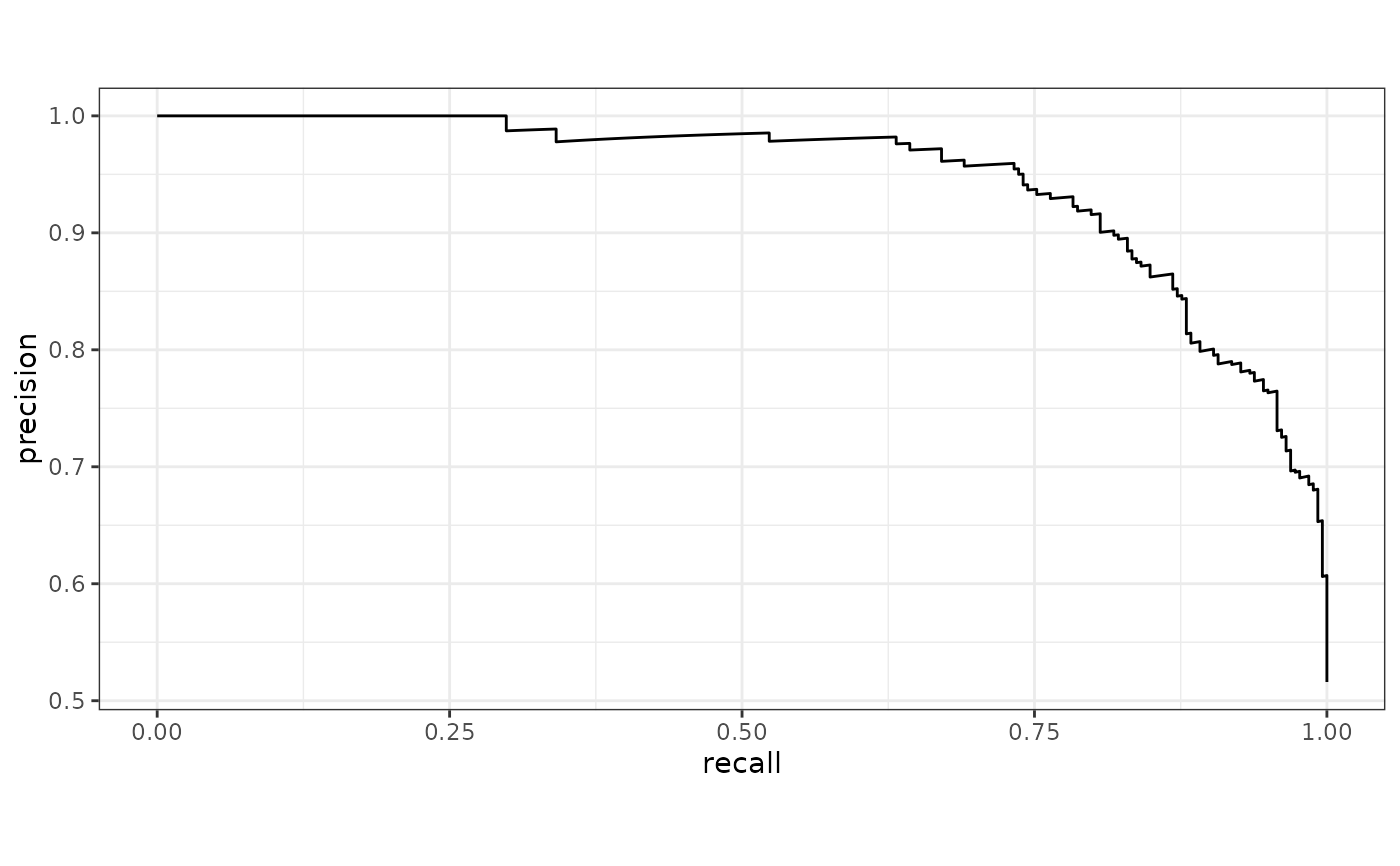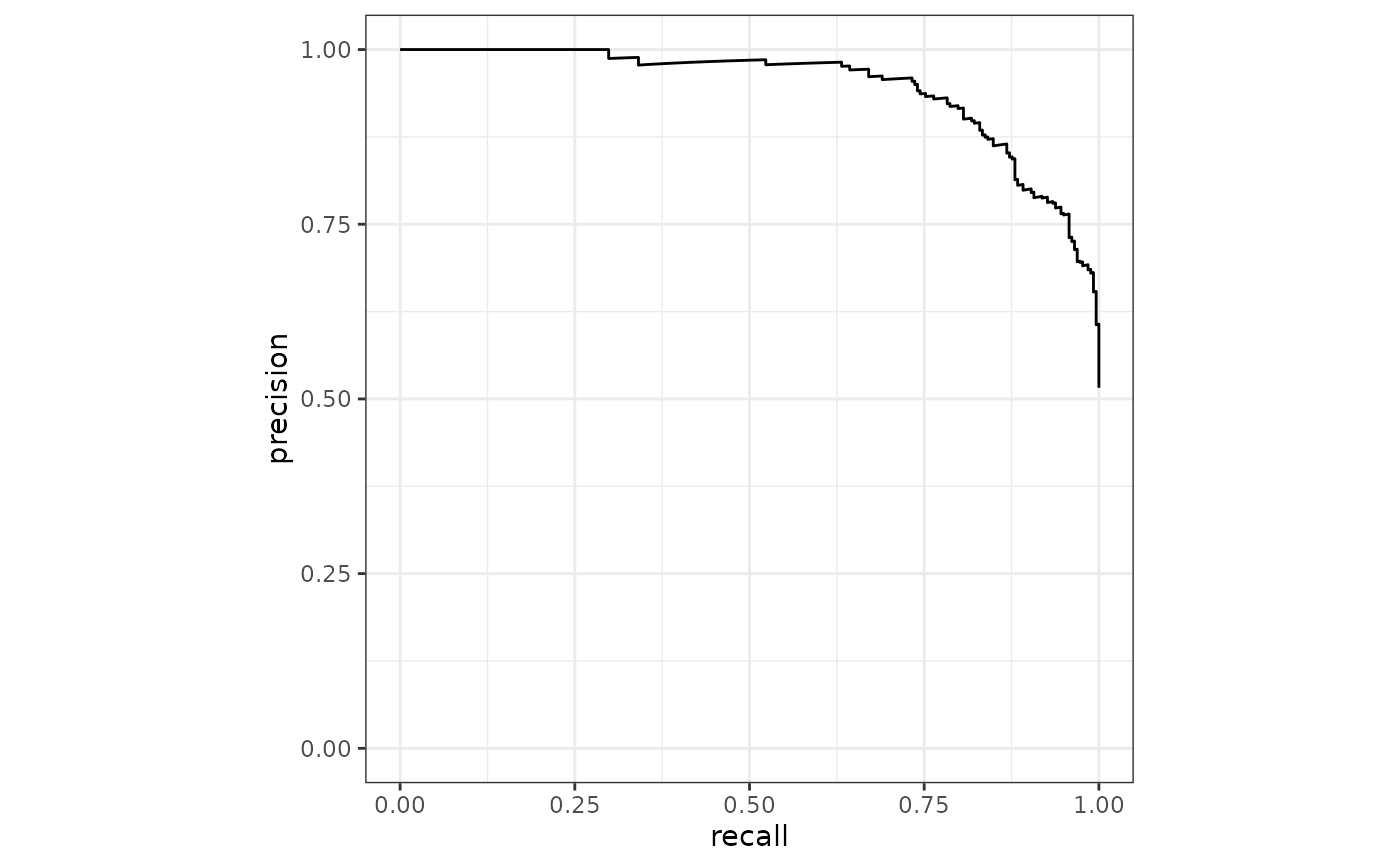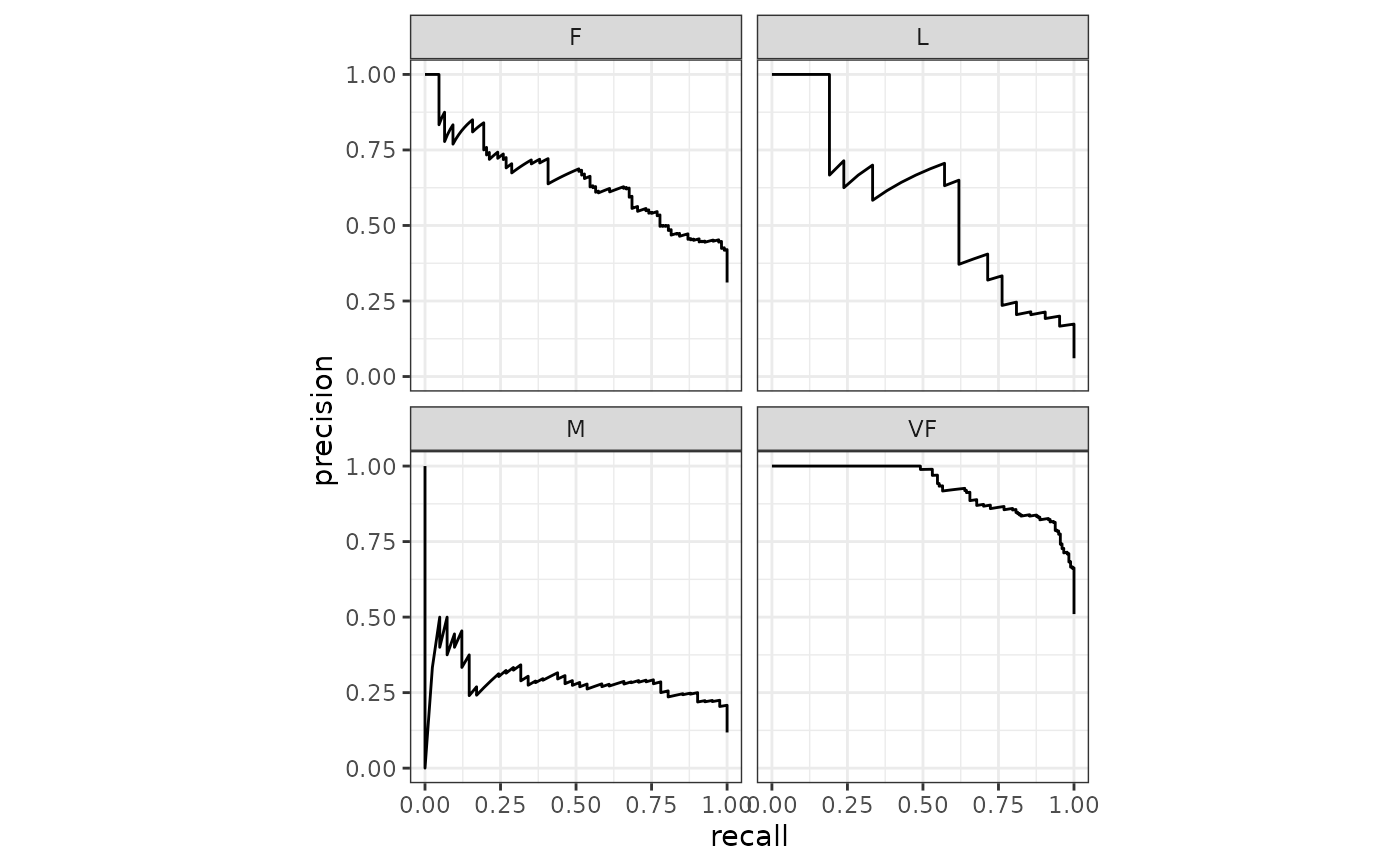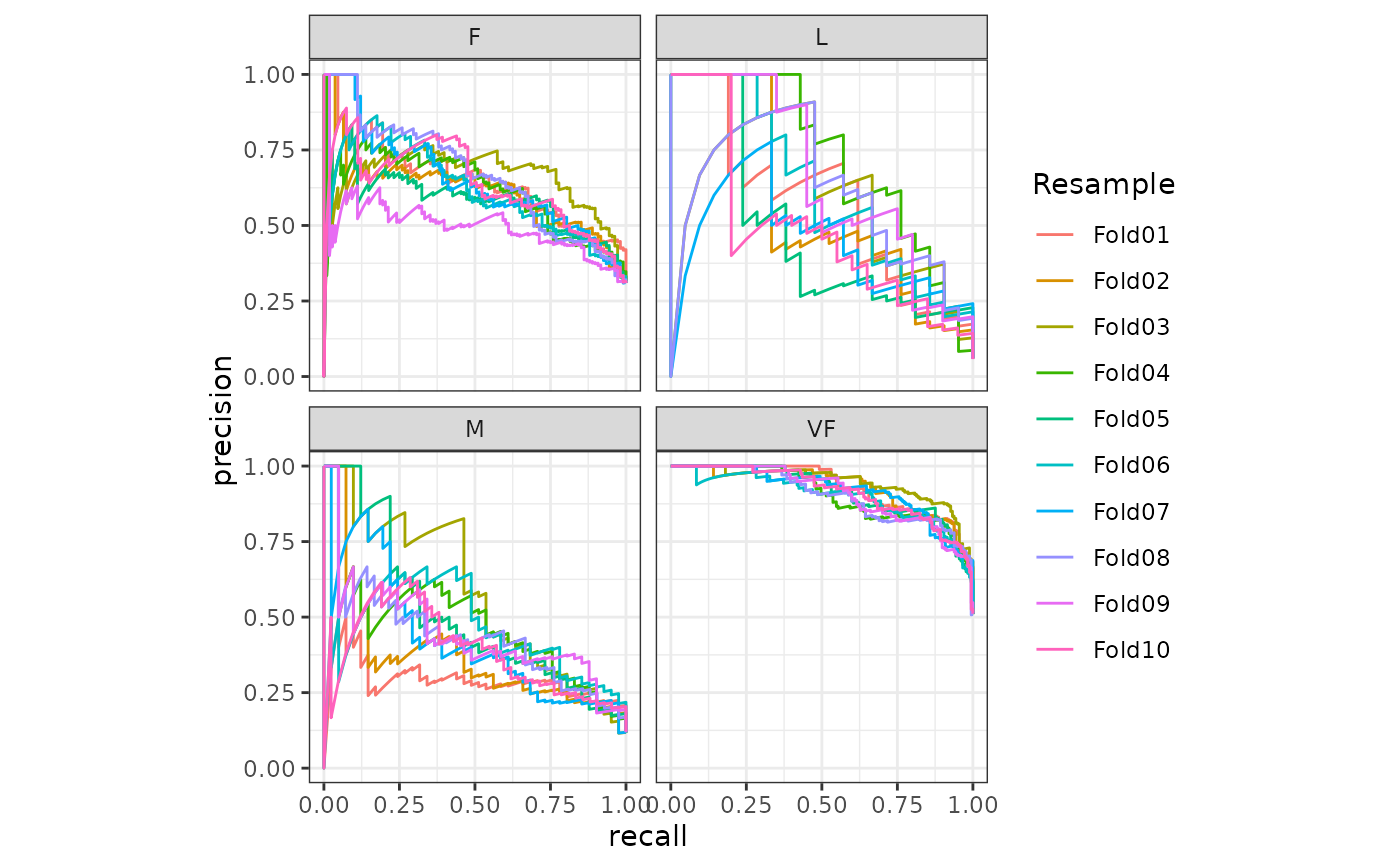pr_curve() constructs the full precision recall curve and returns a tibble. See pr_auc() for the area under the precision recall curve.

## Usage

pr_curve(data, ...)

# S3 method for data.frame
pr_curve(
data,
truth,
...,
na_rm = TRUE,
event_level = yardstick_event_level(),
case_weights = NULL
)

## Arguments

data

A data.frame containing the columns specified by truth and ....

...

A set of unquoted column names or one or more dplyr selector functions to choose which variables contain the class probabilities. If truth is binary, only 1 column should be selected. Otherwise, there should be as many columns as factor levels of truth.

truth

The column identifier for the true class results (that is a factor). This should be an unquoted column name although this argument is passed by expression and supports quasiquotation (you can unquote column names). For _vec() functions, a factor vector.

na_rm

A logical value indicating whether NA values should be stripped before the computation proceeds.

event_level

A single string. Either "first" or "second" to specify which level of truth to consider as the "event". This argument is only applicable when estimator = "binary". The default uses an internal helper that generally defaults to "first", however, if the deprecated global option yardstick.event_first is set, that will be used instead with a warning.

case_weights

The optional column identifier for case weights. This should be an unquoted column name that evaluates to a numeric column in data. For _vec() functions, a numeric vector.

## Value

A tibble with class pr_df or pr_grouped_df having columns .threshold, recall, and precision.

## Details

pr_curve() computes the precision at every unique value of the probability column (in addition to infinity).

There is a ggplot2::autoplot() method for quickly visualizing the curve. This works for binary and multiclass output, and also works with grouped data (i.e. from resamples). See the examples.

## Multiclass

If a multiclass truth column is provided, a one-vs-all approach will be taken to calculate multiple curves, one per level. In this case, there will be an additional column, .level, identifying the "one" column in the one-vs-all calculation.

## Relevant Level

There is no common convention on which factor level should automatically be considered the "event" or "positive" result when computing binary classification metrics. In yardstick, the default is to use the first level. To alter this, change the argument event_level to "second" to consider the last level of the factor the level of interest. For multiclass extensions involving one-vs-all comparisons (such as macro averaging), this option is ignored and the "one" level is always the relevant result.

Compute the area under the precision recall curve with pr_auc().

Other curve metrics: gain_curve(), lift_curve(), roc_curve()

Max Kuhn

## Examples

# ---------------------------------------------------------------------------
# Two class example

# truth is a 2 level factor. The first level is "Class1", which is the
# "event of interest" by default in yardstick. See the Relevant Level
# section above.
data(two_class_example)

# Binary metrics using class probabilities take a factor truth column,
# and a single class probability column containing the probabilities of
# the event of interest. Here, since "Class1" is the first level of
# "truth", it is the event of interest and we pass in probabilities for it.
pr_curve(two_class_example, truth, Class1)
#> # A tibble: 501 × 3
#>    .threshold  recall precision
#>         <dbl>   <dbl>     <dbl>
#>  1     Inf    0               1
#>  2       1.00 0.00388         1
#>  3       1.00 0.00775         1
#>  4       1.00 0.0116          1
#>  5       1.00 0.0155          1
#>  6       1.00 0.0194          1
#>  7       1.00 0.0233          1
#>  8       1.00 0.0271          1
#>  9       1.00 0.0310          1
#> 10       1.00 0.0349          1
#> # … with 491 more rows

# ---------------------------------------------------------------------------
# autoplot()

# Visualize the curve using ggplot2 manually
library(ggplot2)
library(dplyr)
pr_curve(two_class_example, truth, Class1) %>%
ggplot(aes(x = recall, y = precision)) +
geom_path() +
coord_equal() +
theme_bw()# Or use autoplot
autoplot(pr_curve(two_class_example, truth, Class1))# Multiclass one-vs-all approach
# One curve per level
hpc_cv %>%
filter(Resample == "Fold01") %>%
pr_curve(obs, VF:L) %>%
autoplot()# Same as above, but will all of the resamples
hpc_cv %>%
group_by(Resample) %>%
pr_curve(obs, VF:L) %>%
autoplot()